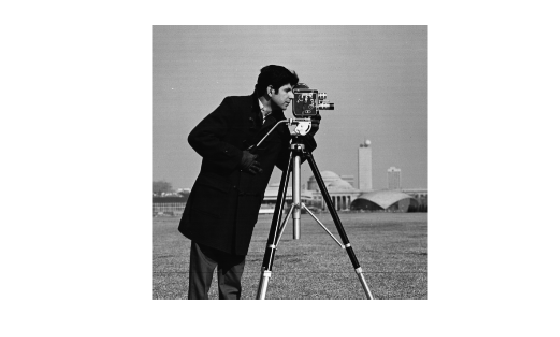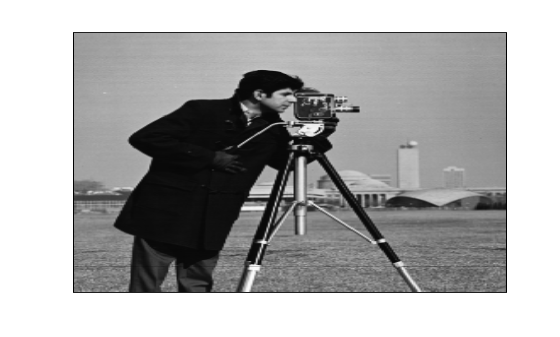geometricTransform2d

2-D geometric transformation object

Description

A geometricTransform2d object defines a custom 2-D geometric transformation using point-wise mapping functions.

Creation

Description

example

tform = geometricTransform2d(inverseFcn) creates a geometricTransform2d object and sets the inverse mapping InverseFcn property.

tform = geometricTransform2d(inverseFcn,forwardFcn) also sets the forward mapping property, ForwardFcn.

Properties

expand all

Inverse mapping function, specified as a function handle. The function should accept and return coordinates as a n-by-2 numeric matrix representing the packed (x,y) coordinates of n points.

Example: ifcn = @(xy) [xy(:,1).^2, sqrt(xy(:,2))];

Forward mapping function, specified as a function handle. The function should accept and return coordinates as a n-by-2 numeric matrix representing the packed (x,y) coordinates of n points.

Example: ffcn = @(xy) [sqrt(xy(:,1)),(xy(:,2).^2)];

Object Functions

 transformPointsForward Apply forward geometric transformation transformPointsInverse Apply inverse geometric transformation

Examples

collapse all

Specify the packed (x,y) coordinates of five input points. The packed coordinates are stored in a 5-by-2 matrix, where the x-coordinate of each point is in the first column, and the y-coordinate of each point is in the second column.

XY = [10 15;11 32;15 34;2 7;2 10];

Define the inverse mapping function. The function accepts and returns points in packed (x,y) format.

inversefn = @(c) [c(:,1)+c(:,2),c(:,1)-c(:,2)]
inversefn = function_handle with value:
@(c)[c(:,1)+c(:,2),c(:,1)-c(:,2)]

Create a 2-D geometric transform object, tform, that stores the inverse mapping function.

tform = geometricTransform2d(inversefn)
tform =
geometricTransform2d with properties:

InverseFcn: @(c)[c(:,1)+c(:,2),c(:,1)-c(:,2)]
ForwardFcn: []
Dimensionality: 2

Apply the inverse geometric transform to the input points.

UV = transformPointsInverse(tform,XY)
UV = 5×2

25    -5
43   -21
49   -19
9    -5
12    -8

Specify the x- and y-coordinates vectors of five points to transform.

x = [10 11 15 2 2];
y = [15 32 34 7 10];

Define the inverse and forward mapping functions. Both functions accept and return points in packed (x,y) format.

inversefn = @(c) [c(:,1).^2,sqrt(c(:,2))];
forwardfn = @(c) [sqrt(c(:,1)),c(:,2).^2];

Create a 2-D geometric transform object, tform, that stores the inverse mapping function and the optional forward mapping function.

tform = geometricTransform2d(inversefn,forwardfn)
tform =
geometricTransform2d with properties:

InverseFcn: @(c)[c(:,1).^2,sqrt(c(:,2))]
ForwardFcn: @(c)[sqrt(c(:,1)),c(:,2).^2]
Dimensionality: 2

Apply the inverse geometric transform to the input points.

[u,v] = transformPointsInverse(tform,x,y)
u = 1×5

100   121   225     4     4

v = 1×5

3.8730    5.6569    5.8310    2.6458    3.1623

Apply the forward geometric transform to the transformed points u and v.

[x,y] = transformPointsForward(tform,u,v)
x = 1×5

10    11    15     2     2

y = 1×5

15.0000   32.0000   34.0000    7.0000   10.0000

Define an inverse mapping function that applies anisotropic scaling. The function must accept and return packed (x,y) coordinates, where the x-coordinate of each point is in the first column, and the y-coordinate of each point is in the second column.

xscale = 0.3;
yscale = 0.5;
inversefn = @(xy) [xscale*xy(:,1), yscale*xy(:,2)];

Create a 2-D geometric transform object, tform, that stores the inverse mapping function.

tform = geometricTransform2d(inversefn)
tform =
geometricTransform2d with properties:

InverseFcn: @(xy)[xscale*xy(:,1),yscale*xy(:,2)]
ForwardFcn: []
Dimensionality: 2

Read an image to be transformed.

imshow(I)Use imwarp to apply the inverse geometric transform to the input image. The image is enlarged vertically by a factor of 2 (the inverse of yscale) and horizontally by a factor of 10/3 (the inverse of xscale).

Itransformed = imwarp(I,tform);
imshow(Itransformed)Define an inverse mapping function that accepts packed (x,y) coordinates, where the x-coordinate of each point is in the first column, and the y-coordinate of each point is in the second column. The inverse mapping function in this example takes the square of the polar radial component.

r = @(c) sqrt(c(:,1).^2 + c(:,2).^2);
w = @(c) atan2(c(:,2), c(:,1));
f = @(c) [r(c).^2 .* cos(w(c)), r(c).^2 .* sin(w(c))];
g = @(c) f(c);

Create a 2-D geometric transform object, tform, that stores the inverse mapping function.

tform = geometricTransform2d(g);

Read a color image to be transformed.

imshow(I)Create an imref2d object, specifying the size and world limits of the input and output images.

Rin = imref2d(size(I),[-1 1],[-1 1]);
Rout = imref2d(size(I),[-1 1],[-1 1]);

Apply the inverse geometric transform to the input image.

Itransformed = imwarp(I,Rin,tform,'OutputView',Rout);
imshow(Itransformed)